In the given figure, when key K is opened, the reading of the ammeter A will be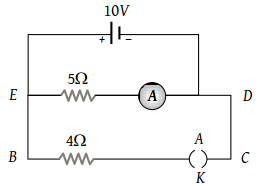(1) 50 A

(2) 2 A

(3) 0.5 A

(4) $\frac{10}{9}A$

Concept Questions :-

Combination of resistors
High Yielding Test Series + Question Bank - NEET 2020

Difficulty Level:

In the given circuit, the potential of the point E is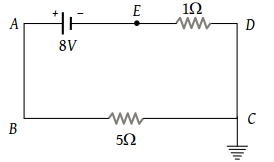(1) Zero

(2) –8 V

(3) –4/3 V

(4) 4/3 V

Concept Questions :-

Kirchoff's voltage law
High Yielding Test Series + Question Bank - NEET 2020

Difficulty Level:

If a resistance R2 is connected in parallel with the resistance R in the circuit shown, then possible value of current through R and the possible value of R2 will be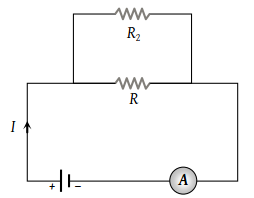(1) $\frac{I}{3},\text{\hspace{0.17em}}R$

(2) $I,\text{\hspace{0.17em}}2R$

(3) $\frac{I}{3},\text{\hspace{0.17em}}R$

(4) $\frac{I}{2},\text{\hspace{0.17em}}R$

Concept Questions :-

Kirchoff's voltage law
High Yielding Test Series + Question Bank - NEET 2020

Difficulty Level:

A battery of e.m.f. 10 V is connected to resistance as shown in figure. The potential difference ${V}_{A}-{V}_{B}$  between the points A and B is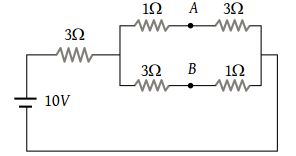(1) –2 V

(2) 2 V

(3) 5 V

(4) $\frac{20}{11}V$

Concept Questions :-

Combination of resistors
High Yielding Test Series + Question Bank - NEET 2020

Difficulty Level:

A student has 10 resistors of resistance ‘r’. The minimum resistance made by him from given resistors is :

(1) 10 r

(2) $\frac{r}{10}$

(3) $\frac{r}{100}$

(4) $\frac{r}{5}$

Concept Questions :-

Combination of resistors
High Yielding Test Series + Question Bank - NEET 2020

Difficulty Level:

Two wires of same metal have the same length but their cross-sections are in the ratio 3 : 1. They are joined in series. The resistance of the thicker wire is 10 Ω. The total resistance of the combination will be

(1) 40 Ω

(2) $\frac{40}{3}\Omega$

(3) $\frac{5}{2}\Omega$

(4) 100 Ω

Concept Questions :-

Combination of resistors
High Yielding Test Series + Question Bank - NEET 2020

Difficulty Level:

The equivalent resistance of the following infinite network of resistances is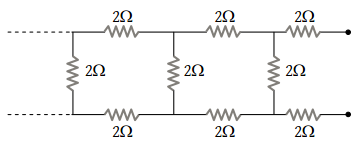(1) Less than 4 Ω

(2) 4 Ω

(3) More than 4 Ω but less than 12 Ω

(4) 12 Ω

Concept Questions :-

Combination of resistors
High Yielding Test Series + Question Bank - NEET 2020

Difficulty Level:

In the figure given below, the current passing through 6 Ω resistor is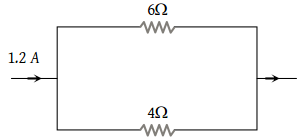(1) 0.40 ampere

(2) 0.48 ampere

(3) 0.72 ampere

(4) 0.80 ampere

Concept Questions :-

Combination of resistors
High Yielding Test Series + Question Bank - NEET 2020

Difficulty Level:

Three equal resistances each of value R are joined as shown in the figure. The equivalent resistance between M and N is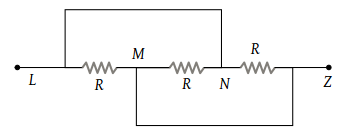(1) R

(2) 2R

(3) $\frac{R}{2}$

(4) $\frac{R}{3}$

Concept Questions :-

Combination of resistors
High Yielding Test Series + Question Bank - NEET 2020

Difficulty Level:

The equivalent resistance between points A and B of an infinite network of resistances each of 1 Ω connected as shown, is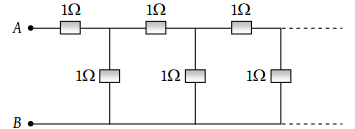(1) Infinite

(2) 2 Ω

(3) $\frac{1+\sqrt{5}}{2}\Omega$

(4) Zero

Concept Questions :-

Combination of resistors